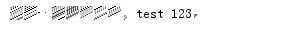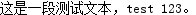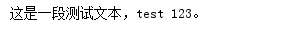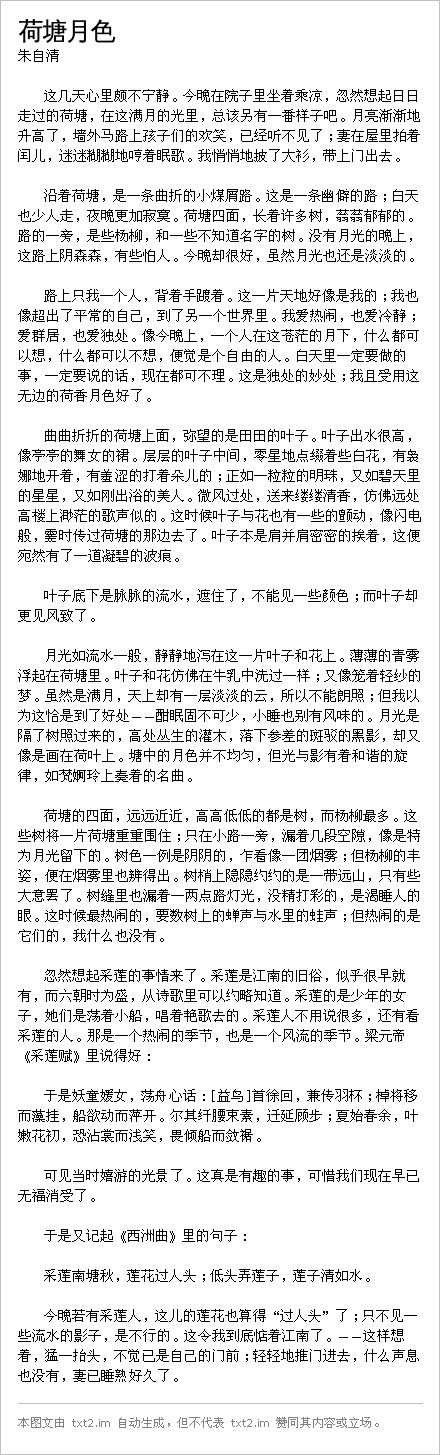# 使用Python脚本将文字转换为图片的实例分享

piaodoo 编程教程 2020-02-02 12:30:48 204 0 python教程

```# -*- coding: utf-8 -*-

import os
import Image, ImageFont, ImageDraw

text = u"这是一段测试文本，test 123。"

im = Image.new("RGB", (300, 50), (255, 255, 255))
dr = ImageDraw.Draw(im)
font = ImageFont.truetype(os.path.join("fonts", "msyh.ttf"), 14)

dr.text((10, 5), text, font=font, fill="#000000")

im.show()
im.save("t.png")
``````font = ImageFont.truetype(os.path.join("fonts", "simsun.ttc"), 18)
```Python 的第三方模块或组件非常多，可用来绘图的除了 PIL 之外，就还有Pycairo、matplotlib、pyGame 等。在这儿，我使用 pyGame 来完成点阵字体的渲染工作。

```# -*- coding: utf-8 -*-

import os
import pygame

pygame.init()

text = u"这是一段测试文本，test 123。"
font = pygame.font.Font(os.path.join("fonts", "simsun.ttc"), 14)
rtext = font.render(text, True, (0, 0, 0), (255, 255, 255))

pygame.image.save(rtext, "t.jpg")
```pyGame 虽然可以解决点阵字体的渲染问题，但讲到对图片的处理，还是 PIL 更为强大。那么，我们为什么不把两者结合起来呢？用 pyGame 渲染点阵字体，然后用 PIL 生成整张图片。

```# -*- coding: utf-8 -*-

import os
import StringIO
import Image, ImageFont, ImageDraw
import pygame

pygame.init()

text = u"这是一段测试文本，test 123。"

im = Image.new("RGB", (300, 50), (255, 255, 255))
#dr = ImageDraw.Draw(im)
#font = ImageFont.truetype(os.path.join("fonts", "simsun.ttc"), 18)
font = pygame.font.Font(os.path.join("fonts", "simsun.ttc"), 14)

#dr.text((10, 5), text, font=font, fill="#000000")
rtext = font.render(text, True, (0, 0, 0), (255, 255, 255))

#pygame.image.save(rtext, "t.gif")
sio = StringIO.StringIO()
pygame.image.save(rtext, sio)
sio.seek(0)

line = Image.open(sio)
im.paste(line, (10, 5))

im.show()
im.save("t.png")
```